# Points

Gryffindor won 437 points. How many points obtained by each of the faculties if they were split at a ratio of 5: 7: 3: 4?

a =  115
b =  161
c =  69
d =  92

### Step-by-step explanation: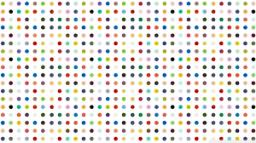Did you find an error or inaccuracy? Feel free to write us. Thank you!

Showing 1 comment:Math student
wrongTips to related online calculators
Need help to calculate sum, simplify or multiply fractions? Try our fraction calculator.
Check out our ratio calculator.

## Related math problems and questions:

• How manyHow many integers are greater than 547/3 and less than 931/4?
• GardenThe rectangular garden has dimensions of 27 m and 30 m. Peter and Katka split it in a ratio of 4:5. How many square meters did Katkin measure part of the garden?
• Change the numbers in the ratio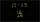Change the numbers 29, 38, and 43 in a 3: 4 ratio.
• BuildingAt the building, we divided 240 boards into two piles in a 5: 3 ratio. How many were fewer boards in the lower pile?
• FruitsAmy bought a basket of fruits 1/5 of them were apples,1/4 were oranges, and the rest were 33 bananas. How many fruits did she buy in all?
• Divide moneyDivide 1200 USD at a ratio of 1:2:3:4:5:6:9:10
• AndreAndre, Thomas, and Ivan split 88 postage stamps in a 2:5:4 ratio. How much did Thomas get?
• Sort fractions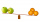Which of the following fractions is the largest? 29/36 5/6 7/9 3/4
• Math testIn mathematics, there were 25 problems of three kinds: light 2 points, medium 3 points, heavy 5 points, the best score is 84 points. How many points did Jane have when she solved all the easy examples, half medium and one-third difficult?
• Fractions and mixed numerals(a) Convert the following mixed numbers to improper fractions. i. 3 5/8 ii. 7 7/6 (b) Convert the following improper fraction to a mixed number. i. 13/4 ii. 78/5 (c) Simplify these fractions to their lowest terms. i. 36/42 ii. 27/45 2. evaluate the follow
• Games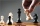Jack and Paul decided to play chess against each other. They bet ten pesos on each game they played. Jack won three bets and Paul won fifty pesos. How many games did they play?
• CompareCompare fractions (34)/(3) and (12)/(4). Which fraction of the lower?
• Money split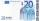Anton and Ferko got 2,500 euros together. The amount was split in a 1:4 ratio. How much did each get?
• Plums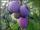In the bag was to total 136 plums. Igor took 3 plums and Mary took 4/7 from the rest. How many plums remained in the bag?
• FlourKim needs 3/4 cup of flour to make 12 cookies. How much flour would she need to make 60 cookies?
• RatiosDivide: a) 250 CZK in the ratio 2:3 b) 1000 CZK in the ratio 4:7:9
• Divisible by 5How many three-digit odd numbers divisible by 5, which are in place ten's number 3?